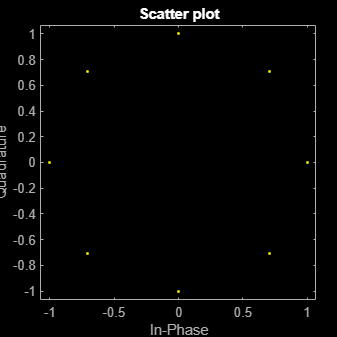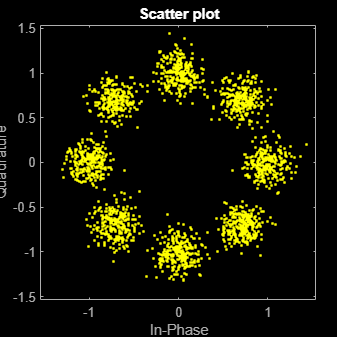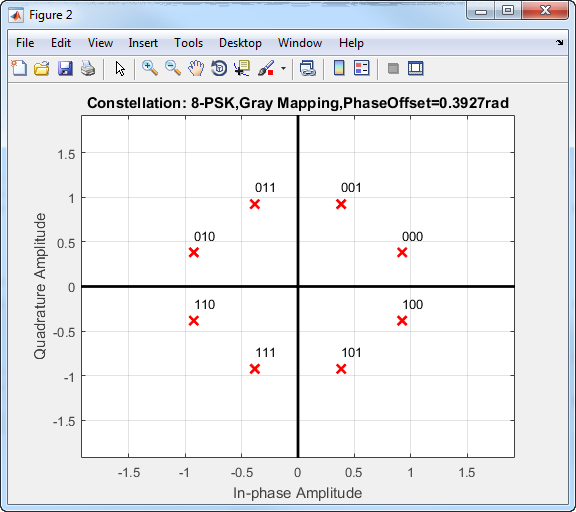Documentation

# comm.PSKModulator

Modulate using M-ary PSK method

## Description

The `PSKModulator` object modulates using the M-ary phase shift keying method. The output is a baseband representation of the modulated signal. The M-ary number parameter, M, is the number of points in the signal constellation.

To modulate a signal using phase shift keying:

1. Define and set up your PSK modulator object. See Construction.

2. Call `step` to modulate the signal according to the properties of `comm.PSKModulator`. The behavior of `step` is specific to each object in the toolbox.

### Note

Starting in R2016b, instead of using the `step` method to perform the operation defined by the System object™, you can call the object with arguments, as if it were a function. For example, ```y = step(obj,x)``` and `y = obj(x)` perform equivalent operations.

## Construction

`H = comm.PSKModulator` creates a modulator System object, `H`. This object modulates the input signal using the M-ary phase shift keying (M-PSK) method.

`H = comm.PSKModulator(Name,Value)` creates an M-PSK modulator object, `H`, with each specified property set to the specified value. You can specify additional name-value pair arguments in any order as (`Name1`,`Value1`,...,`NameN`,`ValueN`).

`H = comm.PSKModulator(M,PHASE,Name,Value)` creates an M-PSK modulator object, `H`. This object has the `ModulationOrder` property set to `M`, the `PhaseOffset` property set to `PHASE`, and the other specified properties set to the specified values.

## Properties

 `ModulationOrder` Number of points in signal constellation Specify the number of points in the signal constellation as a positive, integer scalar value. The default is `8`. `PhaseOffset` Phase of zeroth point of constellation Specify the phase offset of the zeroth point of the constellation, in radians, as a real scalar value. The default is `pi/8`. `BitInput` Assume bit inputs Specify whether the input is bits or integers. When you set this property to `true`, the `step` method input must be a column vector of bit values. This vector must have a length that is an integer multiple of log2(`ModulationOrder`). This vector contains bit representations of integers between `0` and `ModulationOrder`-`1`. When you set the `BitInput` property to `false`, the `step` method input must be a column vector of numeric data type integer symbol values. These values must be between `0` and `ModulationOrder`-`1`. The default is `false`. `SymbolMapping` Constellation encoding Specify how the object maps an integer or group of log2(`ModulationOrder`) input bits to the corresponding symbol as one of `Binary` | `Gray` | `Custom`. The default is `Gray`. When you set this property to `Gray`, the object uses a Gray-encoded signal constellation. When you set this property to `Binary`, the integer m, between `0` $\le m\le$`ModulationOrder`–`1`) maps to the complex value exp(j$×$`PhaseOffset` + j`2`$\pi$m/`ModulationOrder`). When you set this property to `Custom`, the object uses the signal constellation defined in the `CustomSymbolMapping` property. `CustomSymbolMapping` Custom constellation encoding Specify a custom constellation symbol mapping vector. This property requires a row or column vector of size `ModulationOrder` and must have unique integer values in the range [`0`, `ModulationOrder`–`1`]. The values must be of data type `double`. The first element of this vector corresponds to the constellation point at an angle of `0` + `PhaseOffset`, with subsequent elements running counterclockwise. The last element corresponds to the constellation point at an angle of –/`ModulationOrder` + `PhaseOffset`. This property applies when you set the `SymbolMapping` property to `Custom`. The default is `0:7`. `OutputDataType` Data type of output Specify the output data type as `double` | `single` | `Custom`. The default is `double`.

## Methods

 constellation Calculate or plot ideal signal constellation step Modulate using M-ary PSK method
Common to All System Objects
`release`

Allow System object property value changes

## Examples

expand all

Modulate an 8-PSK signal, add white Gaussian noise, and plot the signal to observe the effects of noise.

Create a PSK modulator System object™. The default modulation order for the PSK modulator object is 8.

`pskModulator = comm.PSKModulator;`

Modulate the signal.

`modData = pskModulator(randi([0 7],2000,1));`

Add white Gaussian noise to the modulated signal by passing the signal through an AWGN channel.

`channel = comm.AWGNChannel('EbNo',20,'BitsPerSymbol',3);`

Transmit the signal through the AWGN channel.

`channelOutput = channel(modData);`

Plot the noiseless and noisy data using scatter plots to observe the effects of noise.

`scatterplot(modData)``scatterplot(channelOutput)`Change the `EbNo` property to 10 dB to increase the noise.

`channel.EbNo = 10;`

Pass the modulated data through the AWGN channel.

`channelOutput = channel(modData);`

Plot the channel output. You can see the effects of increased noise.

`scatterplot(channelOutput)`## Algorithms

The block outputs a baseband signal by mapping input bits or integers to complex symbols according to the following:

`${s}_{n}\left(t\right)=\mathrm{exp}\left(j\pi \left(\frac{2n+1}{M}\right)\right);\text{ }n\in \left\{0,1,\dots ,M-1\right\}.$`

This applies when a natural binary ordering is used. Another common mapping is Gray coding, which has the advantage that only one bit changes between adjacent constellation points. This results in better bit error rate performance. For 8-PSK modulation with Gray coding, the mapping between the input and output symbols is shown.

InputOutput
0 0 (000)
1 1 (001)
2 3 (011)
3 2 (010)
4 6 (110)
5 7 (111)
6 5 (101)
7 4 (100)

The corresponding constellation diagram follows.When the input signal is composed of bits, the block accepts binary-valued inputs that represent integers. The block collects binary-valued signals into groups of log2(M) bits.